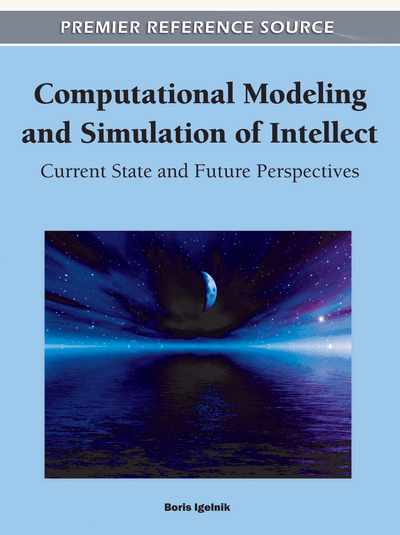Ability of the 1-n-1 Complex-Valued Neural Network to Learn Transformations

Tohru Nitta (National Institute of Advanced Industrial Science and Technology (AIST), Japan)
DOI: 10.4018/978-1-60960-551-3.ch022
Available
\$37.50
No Current Special Offers

Abstract

The ability of the 1-n-1 complex-valued neural network to learn 2D affine transformations has been applied to the estimation of optical flows and the generation of fractal images. The complex-valued neural network has the adaptability and the generalization ability as inherent nature. This is the most different point between the ability of the 1-n-1 complex-valued neural network to learn 2D affine transformations and the standard techniques for 2D affine transformations such as the Fourier descriptor. It is important to clarify the properties of complex-valued neural networks in order to accelerate their practical applications more and more. In this chapter, the behavior of the 1-n-1 complex-valued neural network that has learned a transformation on the Steiner circles is demonstrated, and the relationship the values of the complex-valued weights after training and a linear transformation related to the Steiner circles is clarified via computer simulations. Furthermore, the relationship the weight values of the 1-n-1 complex-valued neural network learned 2D affine transformations and the learning patterns used is elucidated. These research results make it possible to solve complicated problems more simply and efficiently with 1-n-1 complex-valued neural networks. As a matter of fact, an application of the 1-n-1 type complex-valued neural network to an associative memory is presented.
Chapter Preview
Top

Background

Neural Network

A brief overview of neural networks is given.

In the early 1940s, the pioneers of the field, McCulloch and Pitts, proposed a computational model based on a simple neuron-like element (McCulloch & Pitts, 1943). Since then, various types of neurons and neural networks have been developed independently of their direct similarity to biological neural networks. They can now be considered as a powerful branch of present science and technology.

Neurons are the atoms of neural computation. Out of those simple computational neurons all neural networks are build up. An illustration of a (real-valued) neuron is given in Figure 1. The activity of neuron n is defined as:, (1)

where Wnm is the real-valued weight connecting neuron n and m, Xm is the real-valued input signal from neuron m, and Vn is the real-valued threshold value of neuron n. Then, the output of the neuron is given by f(x). Although several types of activation functions f can be used, the most commonly used are the sigmoidal function and the hyperbolic tangent function.

Neural networks can be grouped into two categories: feedforward networks in which graphs have no loops, and recurrent networks where loops occur because of feedback connections. A feedforward type network is made up a certain number of neurons, arranged in layers, and connected with each other through links whose values determine the weight of the connections themselves. Each neuron in a layer is connected to all of the neurons belonging to the following layer and to all of the neurons of the preceding layer. However, there are no weights among neurons in the same layer. The feedforward network can be trained using a certain learning rule to achieve the desired mapping of the input data so as to match the desired target at the network output. The most popular learning rule is the back-propagation learning algorithm (Rumelhart, Hinton, & Williams, 1986). It is well-known that the feedforward neural network can generalize unlearned input data. The characteristic is called the generalization property.

Complete Chapter List

Search this Book:
Reset